Author: Oscar Cronquist Article last updated on February 24, 2022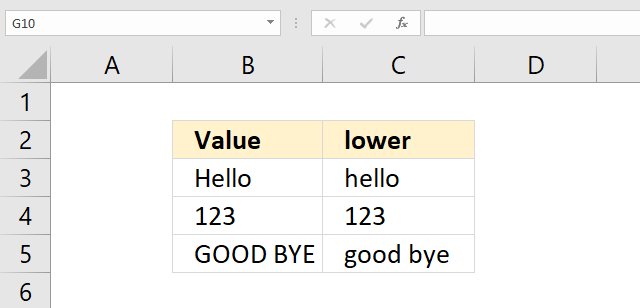The LOWER function converts a value to lower case letters.

Formula in cell C3:

=LOWER(B3)

LOWER(text)

## 2. LOWER Function Arguments

 text Required. The value you want in lower case letters.

## 3. LOWER Function not working

• Check your spelling. Select the cell with the mouse and look for spelling errors in the formula bar.
• Check the number of arguments you use, the UPPER function accepts only one argument. You can, however, use a cell range containing multiple values. You need to enter the formula as an array formula unless you are a Excel 365 user.

## 4. Change upper letter to lower letter based on the position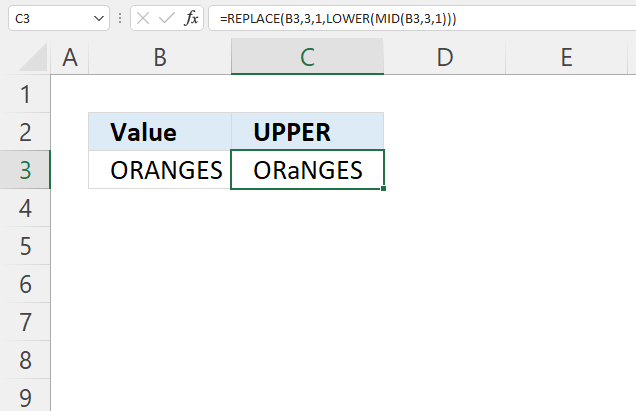The image above shows a formula that substitutes the third letter with a lower case letter.

Formula in cell C3:

=REPLACE(B3, 3, 1, LOWER(MID(B3, 3, 1)))

### 4.1 Explaining formula

#### Step 1 - Extract letter based on position

The MID function returns a substring from a string based on the starting position and the number of characters you want to extract.

MID(textstart_numnum_chars)

MID(B3, 3, 1)

becomes

MID("ORANGES", 3, 1)

and returns "A".

#### Step 2 - Convert to upper letter

The LOWER function converts a string to lower letters.

LOWER(value)

LOWER(MID(B3, 3, 1))

becomes

LOWER("A")

and returns "a".

#### Step 3 - Replace character based on position to an upper letter

The REPLACE function substitutes a string based on character position and length.

REPLACE(old_text, start_num, num_chars, new_text)

REPLACE(B3, 3, 1, LOWER(MID(B3, 3, 1)))

becomes

REPLACE(B3, 3, 1, "a")

becomes

REPLACE("ORANGES", 3, 1, "a")

and returns "ORaNGES".

## 5. Change a given upper letter to a lower letter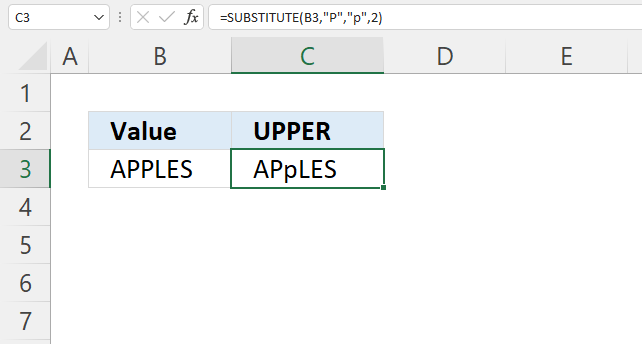The formula in cell C3 demonstrated in the image above converts the second upper case "P" counted from the right with a lower case "p".

Formula in cell C3:

=SUBSTITUTE(B3, "P", "p", 2)

### 5.1 Explaining formula

The SUBSTITUTE function substitutes a string with another string.

SUBSTITUTE(textold_textnew_text, [instance_num])

SUBSTITUTE(B3, "P", "p", 2)

The first argument is a cell reference to cell B3, the second argument is the string to be replaced.

The third argument is what will be there after the substitution and the fourth argument is a number representing the instance.

## 6. Change all upper letters to lower letters for a given character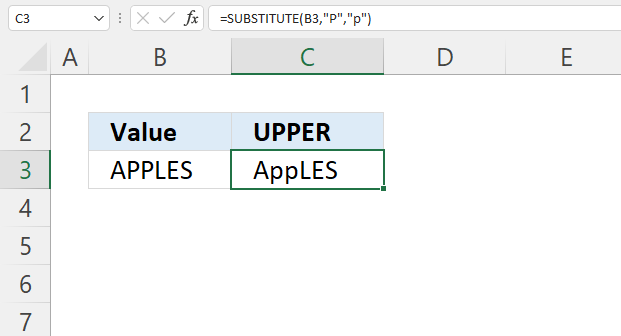The picture above shows a formula in cell C3 that converts all upper case "P" to a lower case "p" in cell B3.

Formula in cell C3:

=SUBSTITUTE(B3, "p", "P")

The SUBSTITUTE function substitutes a string with another string.

SUBSTITUTE(textold_textnew_text, [instance_num])

If the instance_num argument is omitted all instances are replaced.

## 7. Convert every other letter to upper and lower case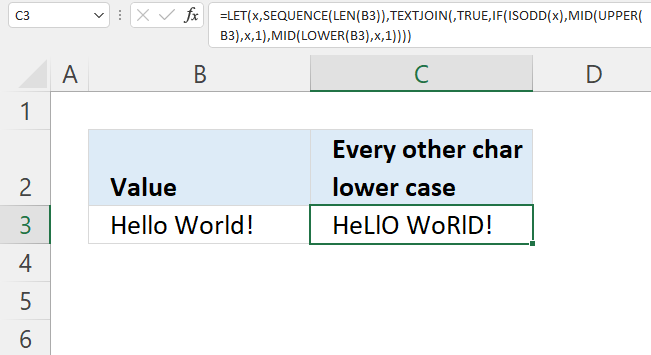Excel 365 formula in cell C3:

=LET(x, SEQUENCE(LEN(B3)), TEXTJOIN(, TRUE, IF(ISODD(x), MID(UPPER(B3), x, 1), MID(LOWER(B3), x, 1))))

### 7.1 Explaining Excel 365 formula

=TEXTJOIN(,TRUE,IF(ISODD(SEQUENCE(LEN(B3))),MID(UPPER(B3),SEQUENCE(LEN(B3)),1),MID(LOWER(B3),SEQUENCE(LEN(B3)),1)))

#### Step 1 - Count charcaters

The LEN function counts the number of characters.

LEN(value)

LEN(B3)

becomes

LEN("Hello World!")

and returns 12. "Hello World!" has twelve characters not counting the double-quotes.

#### Step 2 - Create a sequence of numbers from 1 to n

The SEQUENCE function creates a sequence of numbers.

SEQUENCE(rows, [columns], [start], [step])

SEQUENCE(LEN(B3))

becomes

SEQUENCE(12)

and returns

{1; 2; 3; 4; 5; 6; 7; 8; 9; 10; 11; 12}.

#### Step 3 - Convert string to capital letters

The UPPER function converts a value to upper case letters.

UPPER(value)

UPPER(B3)

becomes

UPPER("Hello World!")

and returns "HELLO WORLD!".

#### Step 4 - Create an array containing each character (upper case)

The MID function returns a substring from a string based on the starting position and the number of characters you want to extract.

MID(textstart_numnum_chars)

MID(UPPER(B3),SEQUENCE(LEN(B3)),1)

becomes

MID("HELLO WORLD!",{1; 2; 3; 4; 5; 6; 7; 8; 9; 10; 11; 12},1)

and returns

{"H"; "E"; "L"; "L"; "O"; " "; "W"; "O"; "R"; "L"; "D"; "!"}.

#### Step 5 - Create an array containing each character (lower case)

MID(LOWER(B3),SEQUENCE(LEN(B3)),1))

becomes

MID("hello world!",{1; 2; 3; 4; 5; 6; 7; 8; 9; 10; 11; 12},1)

and returns

{"h"; "e"; "l"; "l"; "o"; " "; "w"; "o"; "r"; "l"; "d"; "!"}.

#### Step 6 - Identify odd numbers

The ISODD function returns TRUE if a number is odd.

ISODD(number)

ISODD(SEQUENCE(LEN(B3)))

becomes

ISODD({1; 2; 3; 4; 5; 6; 7; 8; 9; 10; 11; 12})

and returns

{TRUE; FALSE; TRUE; FALSE; TRUE; FALSE; TRUE; FALSE; TRUE; FALSE; TRUE; FALSE}.

#### Step 7 - Replace every other character

The IF function returns one value if the logical test is TRUE and another value if the logical test is FALSE.

IF(logical_test, [value_if_true], [value_if_false])

IF(ISODD(SEQUENCE(LEN(B3))), MID(UPPER(B3), SEQUENCE(LEN(B3)), 1), MID(LOWER(B3), SEQUENCE(LEN(B3)), 1))

becomes

IF({TRUE; FALSE; TRUE; FALSE; TRUE; FALSE; TRUE; FALSE; TRUE; FALSE; TRUE; FALSE}, {"H"; "E"; "L"; "L"; "O"; " "; "W"; "O"; "R"; "L"; "D"; "!"}, {"h"; "e"; "l"; "l"; "o"; " "; "w"; "o"; "r"; "l"; "d"; "!"})

and returns

{"H"; "e"; "L"; "l"; "O"; " "; "W"; "o"; "R"; "l"; "D"; "!"}

#### Step 8 - Concatenate characters

The TEXTJOIN function combines text strings from multiple cell ranges and also use delimiting characters if you want.

TEXTJOIN(delimiterignore_emptytext1[text2], ...)

TEXTJOIN(,TRUE,IF(ISODD(SEQUENCE(LEN(B3))), MID(UPPER(B3), SEQUENCE(LEN(B3)), 1), MID(LOWER(B3), SEQUENCE(LEN(B3)), 1)))

becomes

TEXTJOIN(,TRUE,{"H"; "e"; "L"; "l"; "O"; " "; "W"; "o"; "R"; "l"; "D"; "!"})

and returns "HeLlO WoRlD!".

#### Step 9 - Shorten formula

The LET function lets you name intermediate calculation results which can shorten formulas considerably and improve performance.

LET(name1name_value1calculation_or_name2, [name_value2calculation_or_name3...])

SEQUENCE(LEN(B3)) is repeated three times in the formula, lets name this part of the formula x.

LET(x, SEQUENCE(LEN(B3)), TEXTJOIN(, TRUE, IF(ISODD(x), MID(UPPER(B3), x, 1), MID(LOWER(B3), x, 1))))